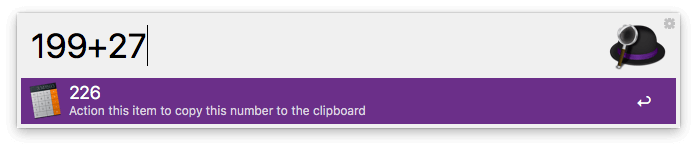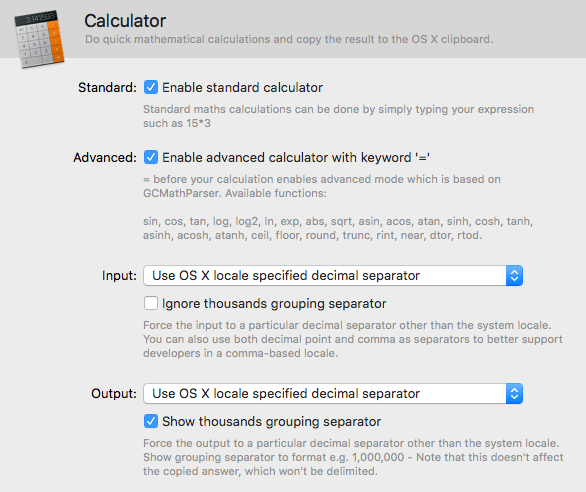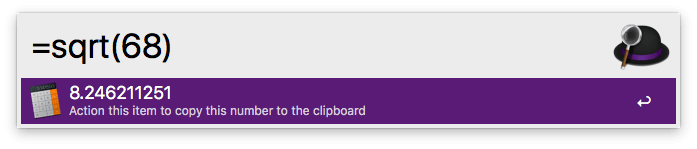Alfred 5 is here - Find out what's new

# Calculator

The calculator is a handy and easy way to do quick maths calculations in Alfred's search box. When you're done, you can copy the result to your clipboard and get on with your work.

## Using the calculator

Alfred knows how to make your life easier in many ways, one of which is helping you with basic maths. Need to do a quick calculation? Simply enter it in the field and the answer will be displayed below.You can also press the = (equal) sign after a calculation to replace your calculation with the solution, making it easy to continue performing further calculations on that result.

## Setting your input and output preferences

In Alfred's preferences under Features > Calculator, you can enable the Standard and Advanced calculators.

You can set your preferences for the input and output format of your calculations. By default, Alfred will use your Mac's locale settings (found in System Preferences > Language & Region > Advanced) but you can force a different preference here.

In other words, you can choose to input or output your calculations with commas or decimal point as decimal separator, and whether results should use thousand separators.Hints & Tips:

If you're making calculations involving currency symbols (Dollar, Pound, Euro signs for example), Alfred will ignore the symbols, so you can paste directly from the web or spreadsheet you copied your figures from without having to remove the dollar signs first!

You can also enable the advanced calculator for more complex maths in Alfred's Features > Calculator preferences.

The advanced calculator uses GCMathParser and can be used by prefixing your calculation with the equal sign (=).You can perform the following calculations with it: `sin`, `cos`, `tan`, `log`, `log2`, `ln`, `exp`, `abs`, `sqrt`, `asin`, `acos`, `atan`, `sinh`, `cosh`, `tanh`, `asinh`, `acosh`, `atanh`, `ceil`, `floor`, `round`, `trunc`, `rint`, `near`, `dtor`, `rtod`

Note: In this mode, `%` is used for the modulo operation.

## Latest Blog Post:

Alfred 5.1.3 Released: Ready for macOS Sonoma